• Predictive Analytics & Forecasting
• Majid Khattak
• NOV 10, 2015

# Modeling Financial Time Series using GARCH

### Introduction

Time series data frequently occurs in finance. The stock price of an asset, the indices of stock exchanges, interest rates and exchange rates are all time series. In this blog I will explore the generalized autoregressive conditional heteroskedastic (GARCH) model. I will show how GARCH model can be fit to a foreign exchange data for the Canadian and the US dollars. The autoregressive conditional heteroskedastic (ARCH) was devised by Robert F. Engle in the early eighties and was later modified to give the GARCH model. Engle won Sveriges Riksbank Prize in Economic Sciences in Memory of Alfred Nobel in 2003.

The data used was obtained from Bank of Italy's website but you may also obtain the forex data from various sources. A simple search on Google will bring up hundreds of websites offering forex data for download for free. My data comprises a daily time series of US dollars and Canadian dollars foreign exchange rates from January 2006 to December 2014. The time series plot of the series is given in the following figure. The "Spot" are the spot prices.

``````#Loading data.
#Converting to ts object.
#Plotting the exchange rate series.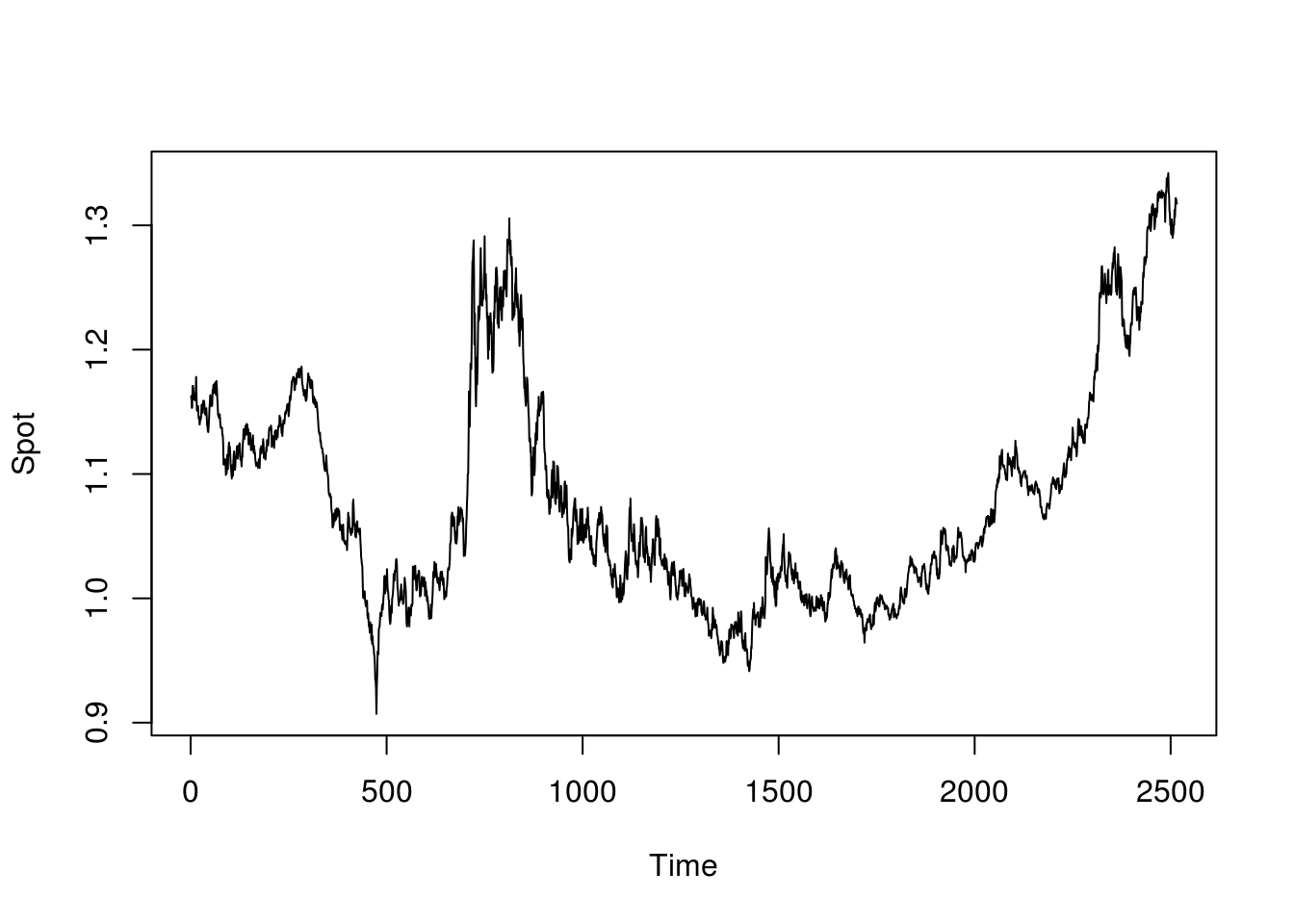When modeling a financial time series, it is better to calculate the returns and use the return series instead of the original series. The reasoning behind using the returns is:

• The returns provide a better and scale-free summary of the data.
• The return series have better statistical properties.

The continuously compounded return is calculated as: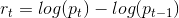where {\(p_t\)} is the value of the time series at time t and log is the natural logarithm. In the following R code, we calculate the continuously compounded return series and then plot this return series.

``````#Calculating the return series
#Plotting the return series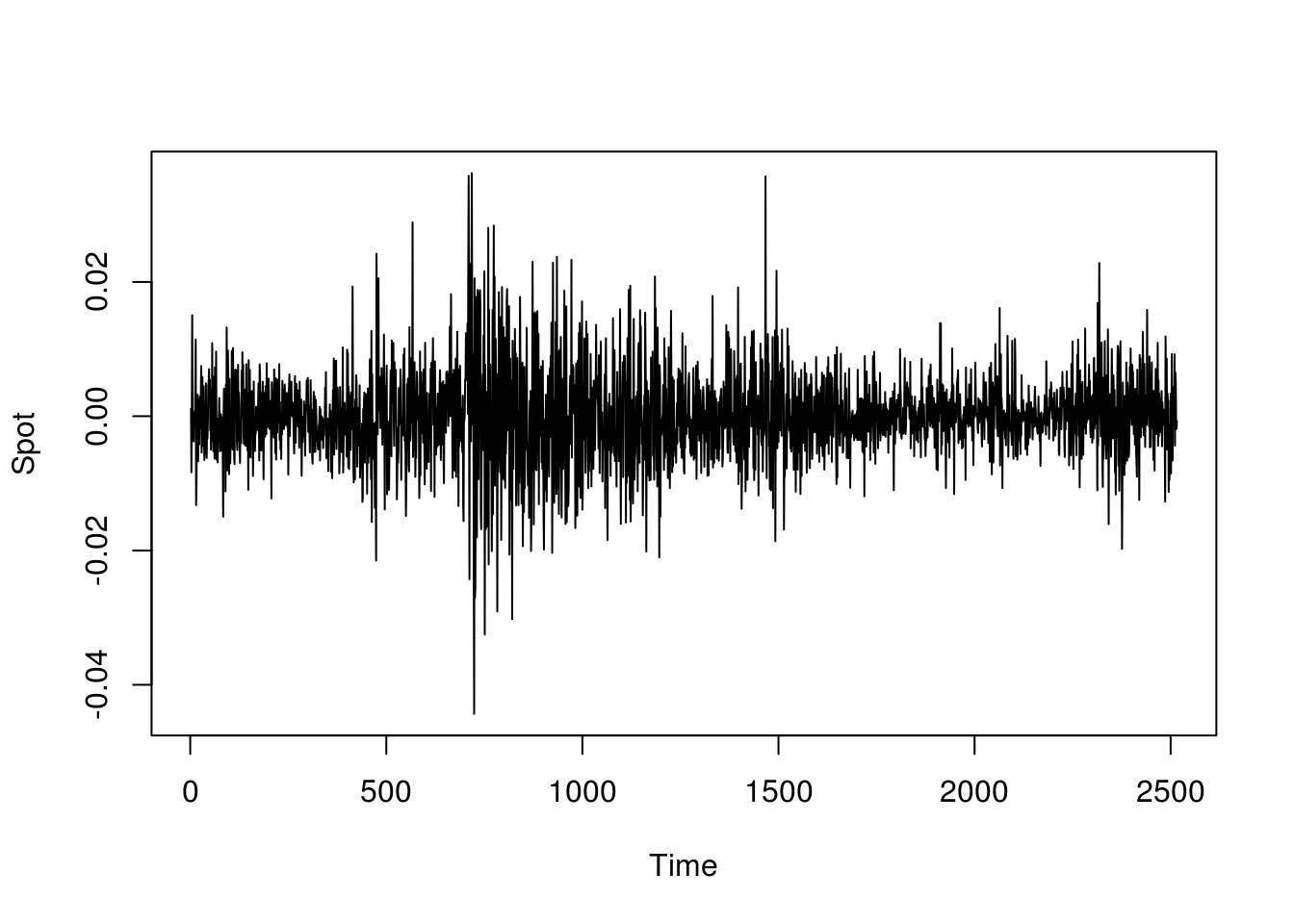The above plot of the return series resembles white noise but on close observation you will note alternating periods of high and low variances. This is called volatility and the series is called conditionally heteroskedastic. To study the series further, we plot the correlogram of the return series.

``acf(usdcadreturn)``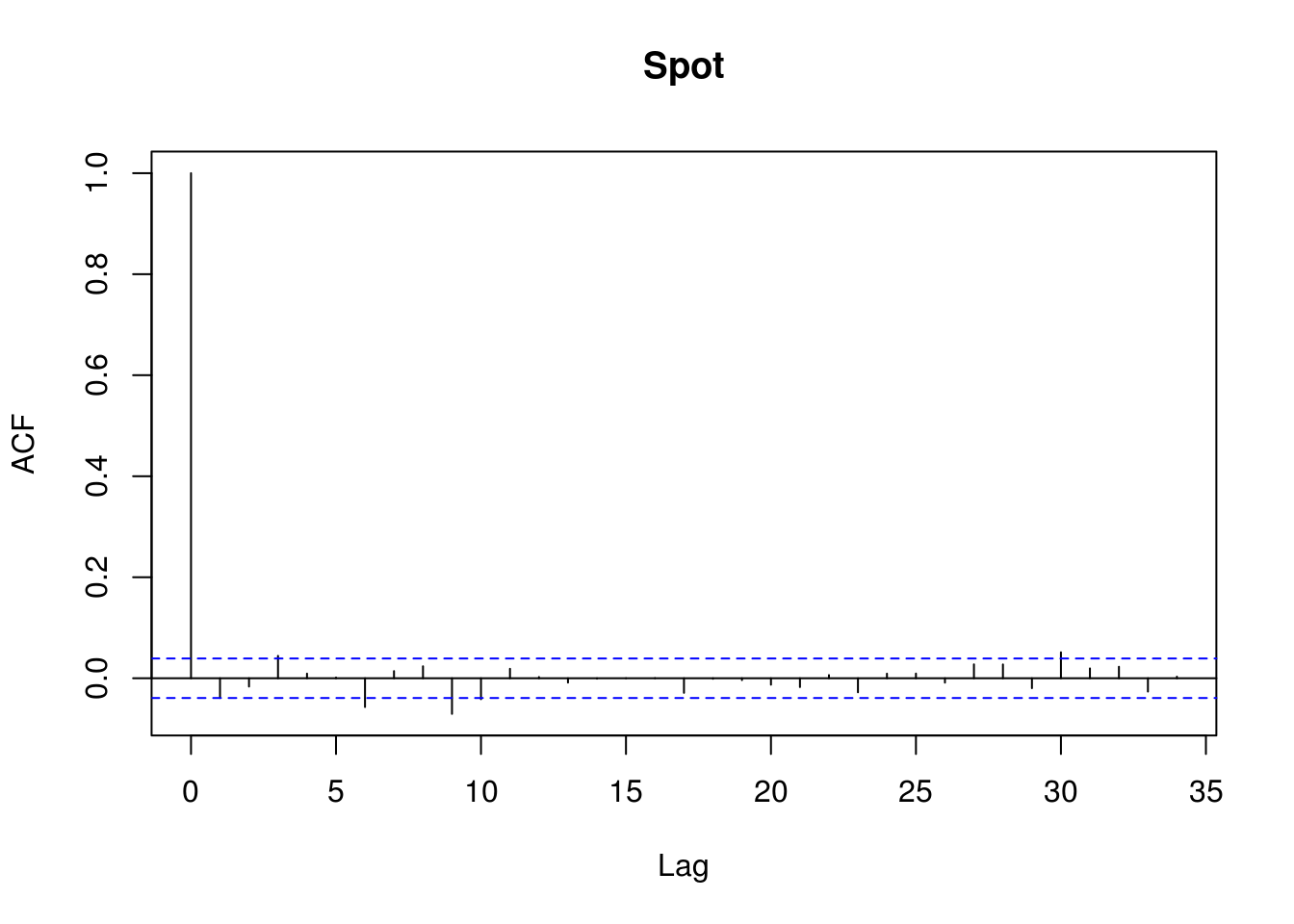Once again the correlogram resembles that of white noise. We will now plot the correlogram of the square of the return series.

``acf(usdcadreturn^2)``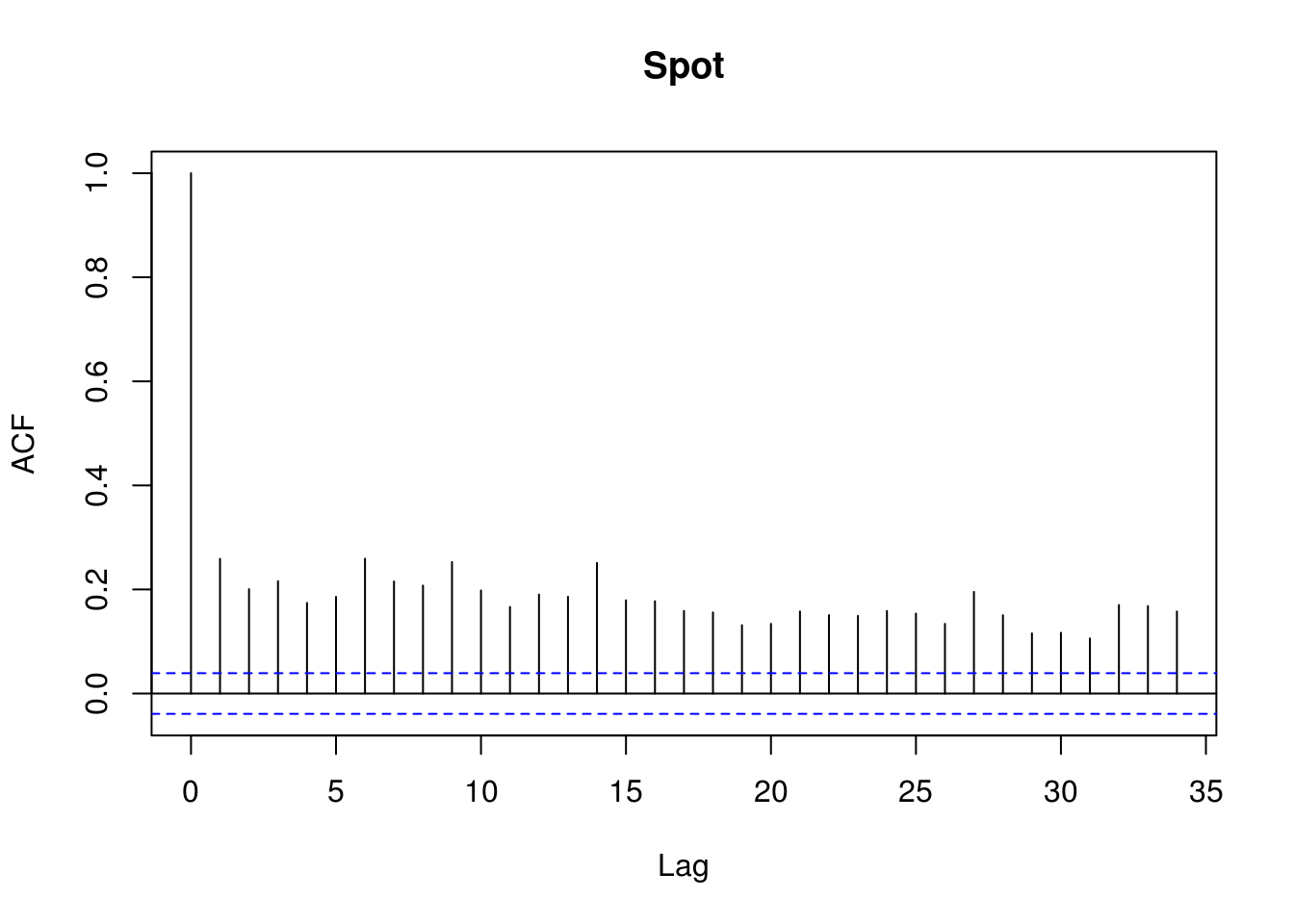Here we see a correlation in the series. The reason for this is that ordinary autocorrelation is a linear measure of the correlation while our series like many financial time series contains a nonlinear correlation.

>

### Fitting the GARCH Model

Mathematically, the GARCH model is expressed as: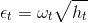where,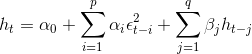and,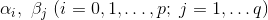are the model parameters.

It can also be written as GARCH(p,q).

I wish the GARCH model was available in the “forecast” package but it is still not bad at all and there is a user-friendly implementation available in the “tseries” R package by Trapletti and Hornik. The following code fits GARCH(1,1) model to our data and displays the coefficients.

``````library(tseries)
#Displaying the coefficients.
``````##
## Call:
## garch(x = usdcadreturn, order = c(1, 1), trace = FALSE)
##
## Coefficient(s):
##        a0         a1         b1
## 1.714e-07  4.863e-02  9.478e-01``````

Plotting the correlogram of the residuals below shows that the model was a good fit.

``acf(ga.usdcadreturn\$res[-1])``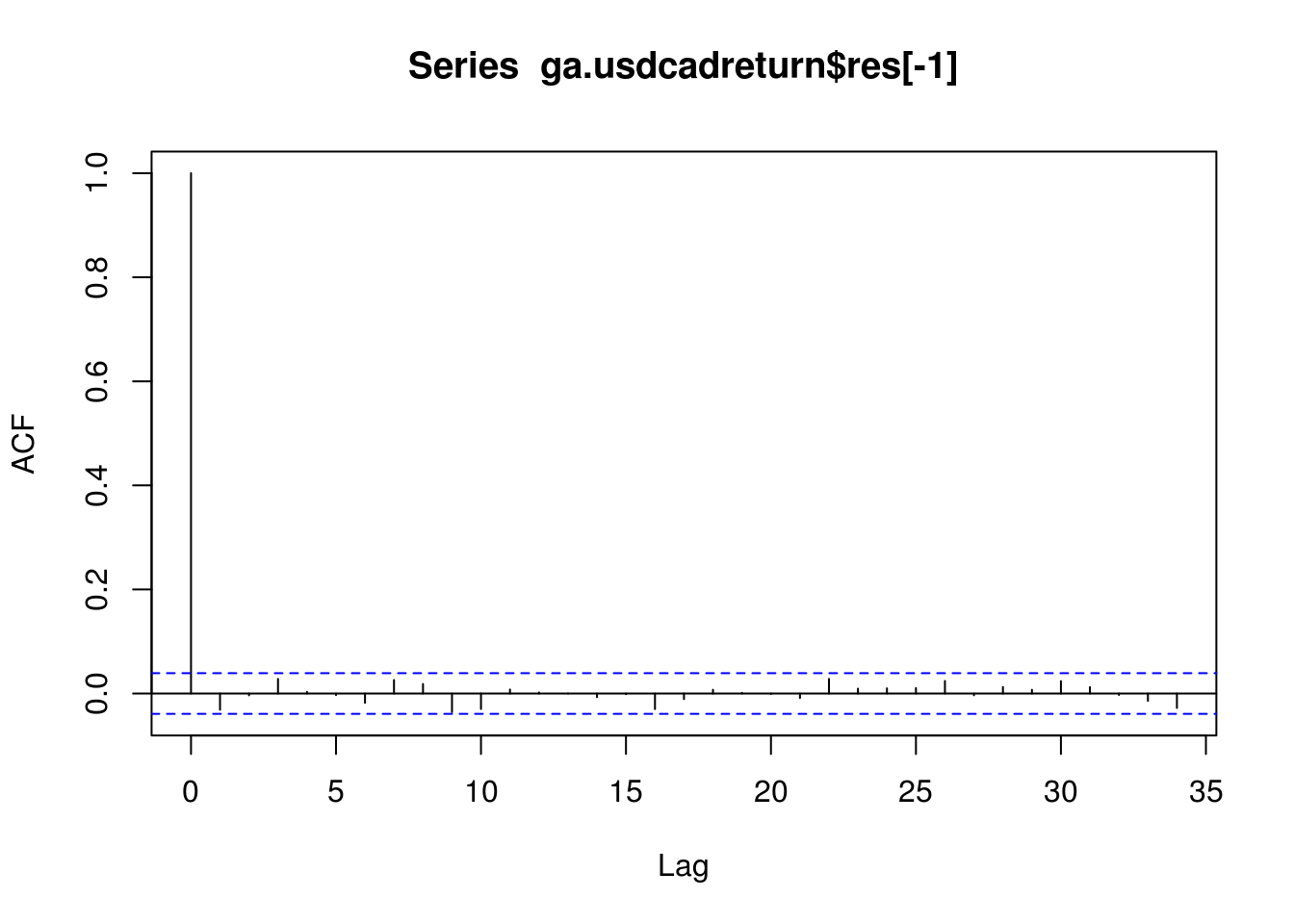### Conclusion

Financial time series are not the only time series where GARCH models are used. They have applications in climate science (where they have been applied to temperature and other climate series), insurance and teletraffic. Anyone interested in learning more about the GARCH models may consult the following books:

• Introductory Time Series with R by Cowpertwiat and Metcalfe (2008).
• Time Series Analysis: With Applications in R by Cryer and Chan (2010).
• Analysis of Financial Time Series by Tsay (2010).